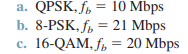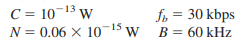Create an Account

Home / Questions / 1 Determine the bandwidth efficiency for the following modulators 2 For the DBPSK modul...

1 Determine the bandwidth efficiency for the following modulators 2 For the DBPSK modulator determine the output phase sequence for the following input bit sequence

1. Determine the bandwidth efficiency for the following modulators:2. For the DBPSK modulator determine the output phase sequence for the following input bit sequence: 00110011010101 (assume that the reference bit 1).

3. For a QPSK system and the given parameters, determine

a. Carrier power in dBm.

b. Noise power in dBm.

c. Noise power density in dBm.

d. Energy per bit in dBJ.

e. Carrier-to-noise power ratio.

f. Eb/Nratio.Jul 28 2020 View more View LessSubscribe To Get Solution